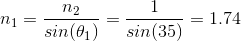# MCAT Physical : Total Internal Reflection

## Example Questions

### Example Question #1 : Total Internal Reflection

A scuba diver wearing a head lamp is returning to the surface of the Pacific Ocean. What is the index of refraction of the ocean water if the smallest angle resulting in total internal reflection is 35 degrees from the vertical?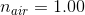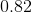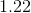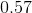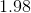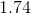Explanation:

We can use Snell's law to calculate the index of refraction of the water: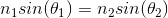Where the subscript 1 denotes water and subscript 2 denotes air.

At the first incidence of total internal reflection, the angle of refraction is 90 degrees. Therefore, the sine function becomes 1, giving us the formula: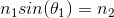Rearranging for the index of water: